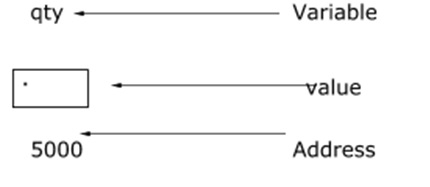# How to calculate sum of array elements using pointers in C language?

Pointer is a variable which stores the address of other variable.

Consider the following statement −

int qty = 179;## Declaring a pointer

The syntax for declaring a pointer is as follows −

int *p;

Here, ‘p’ is a pointer variable which holds the address of other variable.

## Initialization of a pointer

Address operator (&) is used to initialize a pointer variable.

For example,

int qty = 175;
int *p;
p= &qty;

## Array of Pointers

It is collection of addresses (or) collection of pointers.

### Declaration

Following is the declaration for array of pointers −

datatype *pointername [size];

For example,

int *p;

It represents an array of pointers that can hold five integer element addresses.

### Initialization

‘&’ is used for initialization

For example,

int a = {10,20,30};
int *p, i;
for (i=0; i<3; i++) (or) for (i=0; i<3,i++)
p[i] = &a[i];
p[i] = a+i;

### Accessing

Indirection operator (*) is used for accessing.

For example,

for (i=0, i<3; i++)
printf ("%d", *p[i]);

## Program

Following is the C program to calculate the sum of the array elements by using pointers −

Live Demo

//sum of array elements using pointers
#include <stdio.h>
#include <malloc.h>
void main(){
int i, n, sum = 0;
int *ptr;
printf("Enter size of array : ");
scanf("%d", &n);
ptr = (int *) malloc(n * sizeof(int));
printf("Enter elements in the List ");
for (i = 0; i < n; i++){
scanf("%d", ptr + i);
}
//calculate sum of elements
for (i = 0; i < n; i++){
sum = sum + *(ptr + i);
}
printf("Sum of all elements in an array is = %d", sum);
return 0;
}

## Output

When the above program is executed, it produces the following result −

Enter size of array:
5
Enter elements in the List
12
13
14
15
16
Sum of all elements in an array is = 70